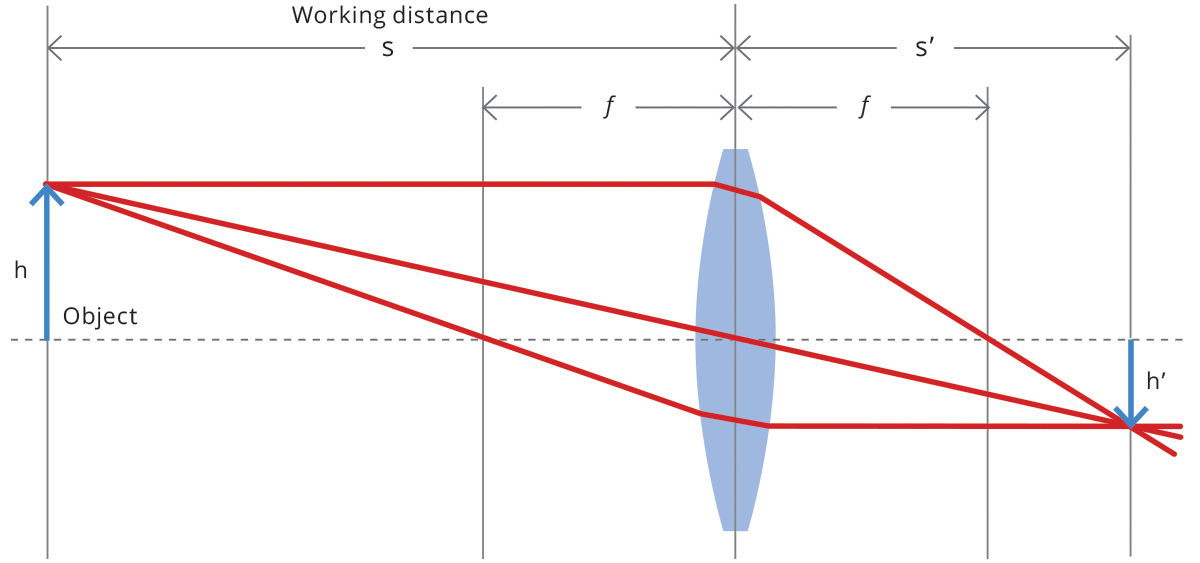## Lens approximations and equations

The main features of most optical systems can be calculated with a few parameters, provided that some approximation is accepted. The paraxial approximation requires that only rays entering the optical system at small angles with respect to the optical axis are taken into account. The thin lens approximation requires the lens thickness to be considerably smaller than the radii of curvature of the lens surfaces: it is thus possible to ignore optical effects due to the real thickness of the lenses and to simplify ray-tracing calculations. Furthermore, assuming that both object and image space are in the same medium (e.g. air), we get the following fundamental equation:

1/s^' - 1/s = 1 / f

where s (s’ ) is the object (image) position with respect to the lens, customarily designated by a negative (positive) value, and f is the focal length of the optical system (cf. Fig. 1). The distance from the object to the front lens is called working distance, while the distance from the rear lens to the sensor is called back focal distance. Henceforth, we will be presenting some useful concepts and formulas based on this simplified model, unless otherwise stated.Next →